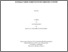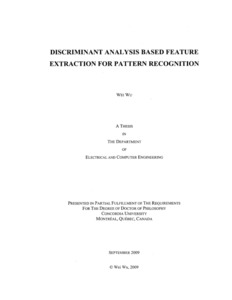Title:

# Discriminant analysis based feature extraction for pattern recognition

Wu, Wei (2009) Discriminant analysis based feature extraction for pattern recognition. PhD thesis, Concordia University.Preview
Text (application/pdf)
NR63403.pdf - Accepted Version
2MB

## Abstract

Fisher's linear discriminant analysis (FLDA) has been widely used in pattern recognition applications. However, this method cannot be applied for solving the pattern recognition problems if the within-class scatter matrix is singular, a condition that occurs when the number of the samples is small relative to the dimension of the samples. This problem is commonly known as the small sample size (SSS) problem and many of the FLDA variants proposed in the past to deal with this problem suffer from excessive computational load because of the high dimensionality of patterns or lose some useful discriminant information. This study is concerned with developing efficient techniques for discriminant analysis of patterns while at the same time overcoming the small sample size problem. With this objective in mind, the work of this research is divided into two parts. In part 1, a technique by solving the problem of generalized singular value decomposition (GSVD) through eigen-decomposition is developed for linear discriminant analysis (LDA). The resulting algorithm referred to as modified GSVD-LDA (MGSVD-LDA) algorithm is thus devoid of the singularity problem of the scatter matrices of the traditional LDA methods. A theorem enunciating certain properties of the discriminant subspace derived by the proposed GSVD-based algorithms is established. It is shown that if the samples of a dataset are linearly independent, then the samples belonging to different classes are linearly separable in the derived discriminant subspace; and thus, the proposed MGSVD-LDA algorithm effectively captures the class structure of datasets with linearly independent samples. Inspired by the results of this theorem that essentially establishes a class separability of linearly independent samples in a specific discriminant subspace, in part 2, a new systematic framework for the pattern recognition of linearly independent samples is developed. Within this framework, a discriminant model, in which the samples of the individual classes of the dataset lie on parallel hyperplanes and project to single distinct points of a discriminant subspace of the underlying input space, is shown to exist. Based on this model, a number of algorithms that are devoid of the SSS problem are developed to obtain this discriminant subspace for datasets with linearly independent samples. For the discriminant analysis of datasets for which the samples are not linearly independent, some of the linear algorithms developed in this thesis are also kernelized. Extensive experiments are conducted throughout this investigation in order to demonstrate the validity and effectiveness of the ideas developed in this study. It is shown through simulation results that the linear and nonlinear algorithms for discriminant analysis developed in this thesis provide superior performance in terms of the recognition accuracy and computational complexity

Divisions: Concordia University > Gina Cody School of Engineering and Computer Science > Electrical and Computer Engineering Thesis (PhD) Wu, Wei xiv, 140 leaves : ill. ; 29 cm. Concordia University Ph. D. Electrical and Computer Engineering 2009 Ahmad, M. Omair 976656 Concordia University Library 22 Jan 2013 16:30 18 Jan 2018 17:42 http://clues.concordia.ca/search/c?SEARC...
All items in Spectrum are protected by copyright, with all rights reserved. The use of items is governed by Spectrum's terms of access.

Repository Staff Only: item control pageResearch related to the current document (at the CORE website)
Back to top